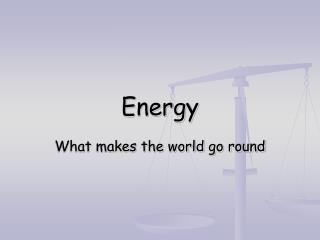Download PresentationEnergy

Loading in 2 Seconds...

# Energy - PowerPoint PPT Presentation

Energy. What makes the world go round. Energy. Stored Energy Energy in Transit. Stored Energy. Potential Kinetic Internal Chemical Nuclear. Potential Energy. PE = W x h W: weight h: height PE = m x g x h m: mass g: acceleration of gravity. Potential Energy. Units: N m = joulesI am the owner, or an agent authorized to act on behalf of the owner, of the copyrighted work described.
Download Presentation## Energy

An Image/Link below is provided (as is) to download presentation

Download Policy: Content on the Website is provided to you AS IS for your information and personal use and may not be sold / licensed / shared on other websites without getting consent from its author.While downloading, if for some reason you are not able to download a presentation, the publisher may have deleted the file from their server.

- - - - - - - - - - - - - - - - - - - - - - - - - - E N D - - - - - - - - - - - - - - - - - - - - - - - - - -
Presentation Transcript
1. Energy What makes the world go round

2. Energy • Stored Energy • Energy in Transit

3. Stored Energy • Potential • Kinetic • Internal • Chemical • Nuclear

4. Potential Energy • PE = W x h • W: weight • h: height • PE = m x g x h • m: mass • g: acceleration of gravity

5. Potential Energy • Units: • N m = joules • Kg m2/s2 = N m = joules • Pounds foot = lb ft

6. Kinetic Energy • Energy of Motion • Kinetic Energy = KE = ½ m v2 • Units: • Kg (m/s)2 = N m = joules • Pounds foot = lb ft

7. Internal Energy • The energy of a body (level of excitement of molecules) • Indicated mainly by temperature • Symbol: U

8. Chemical Energy • Energy released by chemical change • Examples: • Burning coal or other fuel • Body transforming food • Battery

9. Nuclear Energy • Nuclear Fission (splitting atoms) • Nuclear Fusion (combining atoms)

10. Energy in Transit • Burning fuels • Chemical to heat • Electrical resistance • Electrical to heat • Expansion • Heat to mechanical

11. Energy in Transit • Energy required to move an object against resistance, say gravity • This energy defined as work • Work = W = force x distance • Or W = F x d • Where force is in the direction of movement • Units: newton meters or joules

12. Energy in Transit • Heat: • Energy moved from one region to another due to temperature difference • Units: joules • Symbol: Q

13. Conservation of Energy • First Law of Thermodynamics • “Energy can never be created nor destroyed, but only transformed” • Results in Energy Balance: • Energy in system at start + energy added to system = energy in system at end + energy lost from system • Einital + Ein = Efinal + Eout

14. Conservation of Energy • For heat and mechanical work: • Qin + Won + Uinital = Qout + Wby + Ufinal

15. Second Law of Thermodynamics • Not so fast, more rules • Heat can not be converted to work except when it goes from higher to lower temperature • Some processes are not reversible • Friction • Electrical resistance • Heat transfer

16. Thermodynamic Efficiency • If system is reversible then efficiency is 100% • If system is not reversible (all real systems) the efficiency is < 100% • What is maximum possible efficiency? • Carnot efficiency = 1-(T2/T1) • T1 = absolute temperature heat received • T2 = absolute temperature heat rejected

17. Thermodynamic Efficiency • Carnot efficiency assumes no heat or mechanical losses. • For real systems efficiency is • Useful output/total input • Usually expressed as a percentage

18. Thermodynamic Efficiency • Efficiency examples: • Gasoline engines: 17 – 23% • Diesel engines: 26 – 38% • Turbojet aircraft engines 20 – 33% • River Road Power Plant (combine cycle) about 50%

19. Power • Power is the rate at which energy is transferred • Power = W/t or Q/t is a rate • Units: joule/second or watt • Engineering system: BTU/second or horsepower (550 ft lb/s)